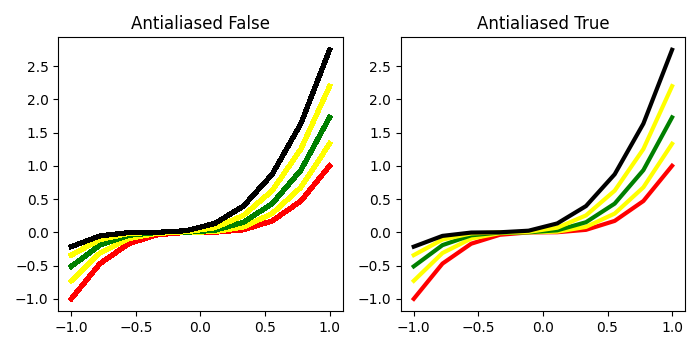# How to change the strength of antialiasing in Matplotlib?

We can change the strength of antialiasing by using True or False flag in the argument of plot() method.

## Steps

• Create x data points and colors list with different colors.

• Defining a method that accepts antialiased flag and axis.

• We can iterate in the range of 5, to print 5 different colors of curves from x data points (Step 1).

• Create a new figure or activate an existing figure.

• Add an axis to the figure as part of a subplot arrangement, at index 1.

• Plot a line with antialiased flag set as False and ax1 (axis 1) and set the title of the figure.

• Add an axis to the figure as part of a subplot arrangement, at index 2.

• Plot a line with antialiased flag set as False and ax2 (axis 2) and set the title of the figure.

• To display the figure, use show() method.

## Example

import numpy as np
from matplotlib import pyplot as plt
plt.rcParams["figure.figsize"] = [7.00, 3.50]
plt.rcParams["figure.autolayout"] = True
x = np.linspace(-1, 1, 10)
colors = ["red", "yellow", "green", "yellow", "black"]
def plot(antialiased, ax):
for i in range(5):
y = (x + i / 10) * (x + i / 10) ** 2
ax.plot(x, y, c=colors[i], lw=3, antialiased=antialiased)
fig = plt.figure()
plot(False, ax1)
ax1.set_title("Antialiased False")
plot(True, ax1)
ax1.set_title("Antialiased True")
plt.show()

## OutputUpdated on: 08-May-2021

463 Views